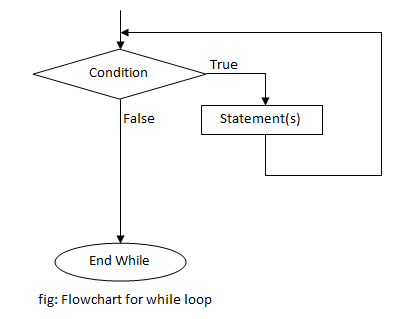# while loop in C Programming

Looping is a process of repeating a certain group of statements until a specified condition is satisfied. There are three types of loop in C. They are:

While loop is an entry controlled loop i.e. the condition is checked before entering into the loop. So if the condition is false for the first time, the statements inside while loop may not be executed at all. The condition to be checked can be changed inside loop by changing values of variables. When the condition becomes false, the program control exits the loop. We can also exit a loop by using break statement like in switch case.

## Syntax of while loop

```while (condition)
{
statement(s);
... ... ...
}```

## Flowchart of while loop## Infinite while loop

If the condition to be tested is always true, the loop will run forever i.e. infinite times. Such conditions should be avoided in programming. For example,

```while (1)
{
printf("This is infinite loop");
}```

This loop will run infinitely. Here, we have kept a non zero value in place of condition so C compiler will treat it as true. To avoid such situation, we shouldn’t use any non zero or non null value in place of condition.

To stop an infinite loop, break statement can be used. For example,

```while (1)
{
printf("This loop will run only once");
break;
}```

## Example of while loop

Example: C program to print the multiplication table of 2 from 1 to 10.

```#include<stdio.h>
int main()
{
int i=1;
while(i<=10)
{
printf("2 * %d = %dn",i,2*i);
i++;
}
return 0;
}```

This program prints a multiplication table of 2 from 1 to 10. We have used while loop to achieve our result. Initially i is assigned to 1. The condition to be tested is i<=10. After executing the loop each time, the value of i is increased by 1. When the value of i becomes 11, the condition becomes false and the loop is terminated.

Output

```2 * 1 = 2
2 * 2 = 4
2 * 3 = 6
2 * 4 = 8
2 * 5 = 10
2 * 6 = 12
2 * 7 = 14
2 * 8 = 16
2 * 9 = 18
2 * 10 = 20```